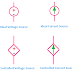Hey, in this article we are going to discuss about the current source and voltage source. Voltage and Current Sources are the type of electrical energy sources to drive the electrical load. Electrical energy sources are active devices and they can be dependent or independent. So here we will discuss the current and voltage source example, properties, types, and difference between them. Remember that Voltage and current source concepts are different from both practical and ideal points of view. Although there are no ideal sources available in the real world but we have to discuss about it for better understanding.

## What is Voltage Source?

An ideal voltage source is an electrical energy source that always delivers or keeps maintain a constant voltage level irrespective of the current delivered by it. Although there is no ideal source available and practical voltage sources never keep maintaining an exactly constant voltage level. There is no short circuit concept in an ideal voltage source. Because if there is a short circuit the voltage across the voltage source will be zero which not supports the definition of an ideal voltage source.

There are maybe different types of voltage sources such as - Ideal Voltage Sources, Practical Voltage sources, Dependent Voltage Sources, Independent Voltage Sources, Controlled Voltage Sources.

## Properties of Voltage Source

1. An ideal voltage source always delivers a constant voltage irrespective of the current drawing from it.

2. An ideal voltage has zero internal resistance, but there is no ideal voltage source available in the real world, so a practical voltage source has some resistance but it is very low compare to load resistance.

3. In a practical voltage source, the terminal voltage always lower than the actual voltage generated by the source due to its internal resistance.

4. In a practical voltage source voltage level changes with current delivering by it.

5. As the voltage source always supplies a constant voltage, so the current drawing from the source depends upon the external circuit or load and any amount of current can be consumed from the voltage source.

6. Voltage drop depends upon the current consumed by the load and the load resistance or circuit component resistance.

## Voltage Source Example

Some examples of voltage source are - Battery(DC Source), Alternator(AC Source), DC Generator(DC Source)

## What is the Current Source?

The current source is an electrical energy source that always a constant current irrespective of the voltage across it or without changing the voltage level. Here also practical and ideal concept available like the voltage source. An ideal current source has infinity internal resistance but practically is not possible. A practical current source has a very high internal resistance but it is finite. A current source always is with a closed-circuit only because if the circuit is open there no current will be flow or the current will be zero.

The current source also be different types such as - Dependent Current Sources, Independent Current Sources, Practical Current Sources, Ideal Current Sources

## Properties of Current Source

1. An ideal current source has infinity internal resistance and a practical current source has a very high internal resistance.

2. The current source is dual of a voltage source.

3. The voltage across the current source is dependent upon the external circuit and load.

4. As the current source always delivers a constant current so the voltage drop or power consumption depends upon the resistance of the load and the circuit component.

## Current Source Examples

Actually, there are no reliable current sources are available. The current source can be made by putting a very high resistance in series with a voltage source. Some examples of Current Sources are - Secondary current in a current transformer, Solar Panels, Semiconductor devices, etc.

In electronics, semiconductor devices are the examples of current source.

## Difference Between Voltage Source and Current Source

 Voltage Source Current Source Always delivers a constant voltage irrespective of current Always delivers a constant current irrespective of voltage It has zero or very low internal resistance It has infinite or very high internal resistance Voltage drop depends upon the load resistance and current Voltage drop depends upon the load resistance Load resistance always higher than internal resistance Load resistance always lower than the internal resistance

Voltage and Current Source Example, Properties, DifferenceReviewed by Author on March 04, 2021 Rating: 5